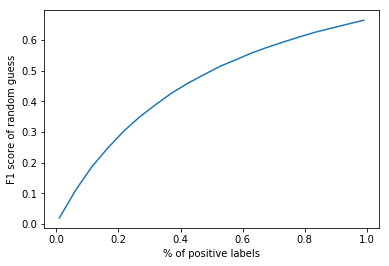#### F1值会受到不平衡数据的影响吗？

统计/机器学习 监督式学习 模型验证    浏览次数：2391        分享

3yangyang   2018-06-14 06:28

##### 2个回答
8from sklearn.metrics import f1_score
import numpy as np
import matplotlib.pyplot as plt

fracs, f1s = [], []
for frac in np.linspace(0.01, 0.99, 20):
n = 1000000
n1 = int(n * frac)
n0 = n - n1
true =  * n1 +  * n0
pred = np.random.randint(0, 2, n)
fracs.append(frac)
f1s.append(f1_score(true, pred))

plt.plot(fracs, f1s)
plt.xlabel('% of positive labels')
plt.ylabel('F1 score of random guess')
plt.show()

SofaSofa数据科学社区DS面试题库 DS面经mrhust   2018-06-21 23:11

4

SofaSofa数据科学社区DS面试题库 DS面经Nagozi   2018-06-25 05:48相关主题